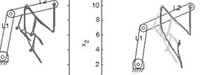### Abstract

We present a probabilistic architecture for solving generically the problem of extracting the task constraints through a Programming by Demonstration (PbD) framework and for generalizing the acquired knowledge to various situations. In previous work, we proposed an approach based on Gaussian Mixture Regression (GMR) to find a controller for the robot reproducing the statistical characteristics of a movement in joint space and in task space through Lagrange optimization. In this paper, we develop an alternative procedure to handle simultaneously constraints in joint space and in task space by combining directly the probabilistic representation of the task constraints with a solution to Jacobian-based inverse kinematics. The method is validated in manipulation tasks with two 5 DOFs Katana robotic arms displacing a set of objects.

### Bibtex reference

@article{Calinon09AR,
author="S. Calinon and A. Billard",
title="Statistical Learning by Imitation of Competing Constraints in Joint Space and Task Space",
journal="Advanced Robotics",
year="2009",
publisher="The Robotics Society of Japan",
address="Tokyo, Japan",
volume="23",
number="",
pages="2059--2076",
}

### Video

Incremental learning of a motion with two Katana robotic arms from Neuronics.
Two demonstrations are provided to show to the robots how to pour some liquid from a bottle into a glass. Then, the user moves manually one of the robot while the other robot follows as best as possible the learned motion in a flexible manner.

Video credit: Florent D'halluin.

### Source codes

#### DownloadDownload Joint/task constraints sourcecode

#### Usage

Unzip the file and run 'demo1' in Matlab.

#### Reference

• Calinon, S. and Billard, A. (2008) A Probabilistic Programming by Demonstration Framework Handling Constraints in Joint Space and Task Space. IEEE/RSJ International Conference on Intelligent Robots and Systems (IROS), pp. 367-372.

#### Demo 1 - Demonstration of the use of Gaussian Mixture Regression (GMR) and inverse kinematics to reproduce a task by considering constraints both in joint space and in task spaceThis program shows the simulation of a robotic arm composed of 2 links and moving in 2D space. Several demonstrations of a skill are provided, by starting from different initial positions. The skill consists of moving each joint sequentially and then writing the alphabet letter 'N' at a specific position in the 2D space.
Constraints in joint space and in task space are represented through Gaussian Mixture Models (GMMs) and Gaussian Mixture Regression (GMR). By using an inverse kinematics process based on a pseudo-inverse Jacobian, the constraints in task space are then projected in joint space. By considering the projected constraints with the ones originally encoded in joint space, an optimal controller is found for the reproduction of the task. We see through this example that the system is able to generalize the learned skill to new robotic arms (different links lengths) and to new initial positions of the robot.